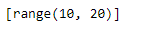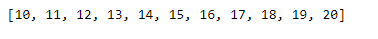# range() to a list in Python

Often times we want to create a list containing a continuous value like, in a range of 100-200. Let’s discuss how to create a list using the `range()` function.

Will this work ?

 `# Create a list in a range of 10-20 ` `My_list ``=` `[``range``(``10``, ``20``, ``1``)] ` ` `  `# Print the list ` `print``(My_list) `

Output :As we can see in the output, the result is not exactly what we were expecting because Python does not unpack the result of the range() function.

Code #1: We can use argument-unpacking operator i.e. *.

 `# Create a list in a range of 10-20 ` `My_list ``=` `[``*``range``(``10``, ``21``, ``1``)] ` ` `  `# Print the list ` `print``(My_list) `

Output :As we can see in the output, the argument-unpacking operator has successfully unpacked the result of the range function.

Code #2 : We can use the `extend()` function to unpack the result of range function.

 `# Create an empty list ` `My_list ``=` `[] ` ` `  `# Value to begin and end with ` `start, end ``=` `10``, ``20` ` `  `# Check if start value is smaller than end value ` `if` `start < end: ` `    ``# unpack the result ` `    ``My_list.extend(``range``(start, end)) ` `    ``# Append the last value ` `    ``My_list.append(end) ` ` `  `# Print the list ` `print``(My_list) `

Output :Whether you're preparing for your first job interview or aiming to upskill in this ever-evolving tech landscape, GeeksforGeeks Courses are your key to success. We provide top-quality content at affordable prices, all geared towards accelerating your growth in a time-bound manner. Join the millions we've already empowered, and we're here to do the same for you. Don't miss out - check it out now!

Previous
Next